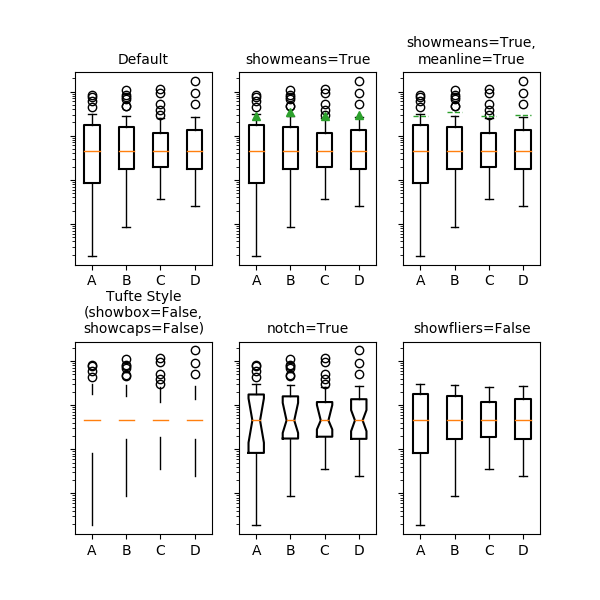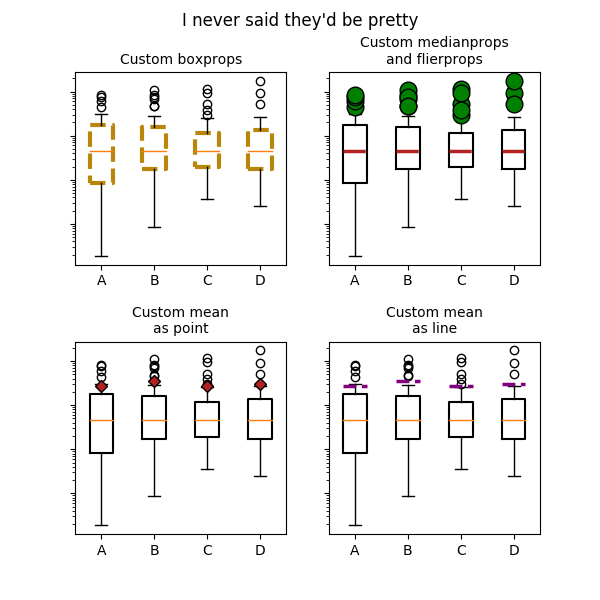# matplotlib.axes.Axes.bxp¶

Axes.bxp(bxpstats, positions=None, widths=None, vert=True, patch_artist=False, shownotches=False, showmeans=False, showcaps=True, showbox=True, showfliers=True, boxprops=None, whiskerprops=None, flierprops=None, medianprops=None, capprops=None, meanprops=None, meanline=False, manage_xticks=True, zorder=None)[source]

Drawing function for box and whisker plots.

Make a box and whisker plot for each column of x or each vector in sequence x. The box extends from the lower to upper quartile values of the data, with a line at the median. The whiskers extend from the box to show the range of the data. Flier points are those past the end of the whiskers.

Parameters: bxpstats : list of dicts A list of dictionaries containing stats for each boxplot. Required keys are: med: The median (scalar float). q1: The first quartile (25th percentile) (scalar float). q3: The third quartile (75th percentile) (scalar float). whislo: Lower bound of the lower whisker (scalar float). whishi: Upper bound of the upper whisker (scalar float). Optional keys are: mean: The mean (scalar float). Needed if showmeans=True. fliers: Data beyond the whiskers (sequence of floats). Needed if showfliers=True. cilo & cihi: Lower and upper confidence intervals about the median. Needed if shownotches=True. label: Name of the dataset (string). If available, this will be used a tick label for the boxplot positions : array-like, default = [1, 2, ..., n] Sets the positions of the boxes. The ticks and limits are automatically set to match the positions. widths : array-like, default = None Either a scalar or a vector and sets the width of each box. The default is 0.15*(distance between extreme positions), clipped to no less than 0.15 and no more than 0.5. vert : bool, default = False If True (default), makes the boxes vertical. If False, makes horizontal boxes. patch_artist : bool, default = False If False produces boxes with the Line2D artist. If True produces boxes with the Patch artist. shownotches : bool, default = False If False (default), produces a rectangular box plot. If True, will produce a notched box plot showmeans : bool, default = False If True, will toggle on the rendering of the means showcaps : bool, default = True If True, will toggle on the rendering of the caps showbox : bool, default = True If True, will toggle on the rendering of the box showfliers : bool, default = True If True, will toggle on the rendering of the fliers boxprops : dict or None (default) If provided, will set the plotting style of the boxes whiskerprops : dict or None (default) If provided, will set the plotting style of the whiskers capprops : dict or None (default) If provided, will set the plotting style of the caps flierprops : dict or None (default) If provided will set the plotting style of the fliers medianprops : dict or None (default) If provided, will set the plotting style of the medians meanprops : dict or None (default) If provided, will set the plotting style of the means meanline : bool, default = False If True (and showmeans is True), will try to render the mean as a line spanning the full width of the box according to meanprops. Not recommended if shownotches is also True. Otherwise, means will be shown as points. manage_xticks : bool, default = True If the function should adjust the xlim and xtick locations. zorder : scalar, default = None The zorder of the resulting boxplot result : dict A dictionary mapping each component of the boxplot to a list of the matplotlib.lines.Line2D instances created. That dictionary has the following keys (assuming vertical boxplots): boxes: the main body of the boxplot showing the quartiles and the median's confidence intervals if enabled. medians: horizontal lines at the median of each box. whiskers: the vertical lines extending to the most extreme, non-outlier data points. caps: the horizontal lines at the ends of the whiskers. fliers: points representing data that extend beyond the whiskers (fliers). means: points or lines representing the means.

Examples(png, pdf)